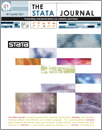Home  >>  Archives  >>  Volume 14 Number 4  >>  gr0059

#### The Stata JournalVolume 14 Number 4: pp. 708-737

Subscribe to the Stata Journal## Plotting regression coefficients and other estimates

 Ben Jann University of Bern Bern, Switzerland jann@soz.unibe.ch
Abstract.  Graphical display of regression results has become increasingly popular in presentations and in scientific literature because graphs are often much easier to read than tables. Such plots can be produced in Stata by the marginsplot command (see [R] marginsplot). However, while marginsplot is versatile and flexible, it has two major limitations: it can only process results left behind by margins (see [R] margins), and it can handle only one set of results at a time. In this article, I introduce a new command called coefplot that overcomes these limitations. It plots results from any estimation command and combines results from several models into one graph. The default behavior of coefplot is to plot markers for coefficients and horizontal spikes for confidence intervals. However, coefplot can also produce other types of graphs. I illustrate the capabilities of coefplot by using a series of examples.

View all articles by this author: Ben Jann

View all articles with these keywords: coefplot, marginsplot, margins, regression plot, coefficients plot, ropeladder plot